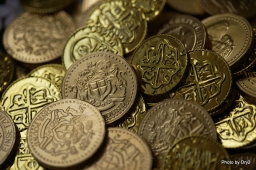# Grandmother 9451

Petr and Honza received 315 CZK from their grandmother. Petr Dostál a third more than Honza. How many crowns did each of them have?

h =  135
p =  180

### Step-by-step explanation:

p+h=315
p = h + h/3

h+p = 315
4h-3p = 0

Pivot: Row 1 ↔ Row 2
4h-3p = 0
h+p = 315

Row 2 - 1/4 · Row 1 → Row 2
4h-3p = 0
1.75p = 315

p = 315/1.75 = 180
h = 0+3p/4 = 0+3 · 180/4 = 135

h = 135
p = 180

Our linear equations calculator calculates it.Did you find an error or inaccuracy? Feel free to write us. Thank you!

Tips for related online calculators
Need help calculating sum, simplifying, or multiplying fractions? Try our fraction calculator.
Do you have a linear equation or system of equations and looking for its solution? Or do you have a quadratic equation?